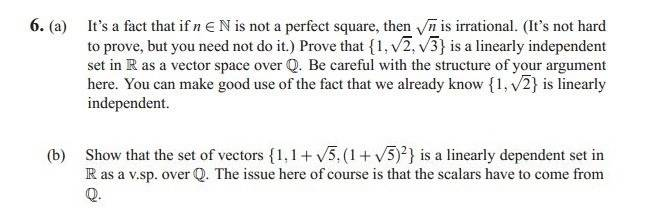# Linear independence

stripes

## Homework Statement## The Attempt at a Solution

For part (a):

a*1 + b*√2 + c*√3 = 0
assume a, b, c not all zero
a + b√2 = -c√3
a2 + 2b2 + 2ab√2 = 3c2
a2 + 2b2 - 3c2 = -2ab√2
(a2 + 2b2 - 3c2)/(-2ab) = √2

which is not possible since we take a, b, c to be rational, and √2 is irrational.

thus our assumption of a, b, c not all zero was false and we must have a=b=c=0.

For part (b), a similar argument, but easier:

{1, 1 + √5, (1 + √5)2} = {1, 1 + √5, 1 + 2√5 + 5}

a + b(1 + √5) + c(1 + 2√5 + 5) = 0
assume a, b, c not all zero
1 + b + b√5 + c + 2c√5 + 5c = 0
1 + b + c + 5c = -b√5 - 2c√5
1 + b + 6c = (-b - 2c)√5
(1 + b + 6c)/(-b - 2c) = √5

same story as before.

Now that was super easy. And the assignment says to be careful with the structure of my argument. And I hate denominators because they can't be zero. If a = b = c = 0, then we have 0/0, which is of indeterminate form, which is ok! but i'm getting the idea that my argument is flawed because of division by zero...